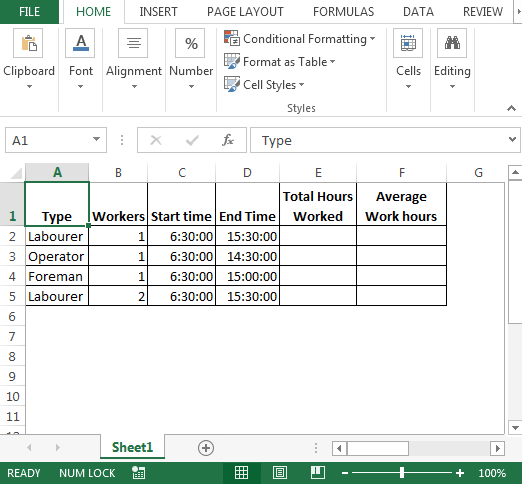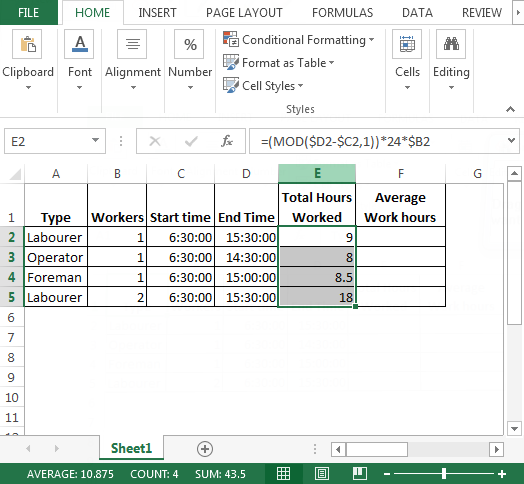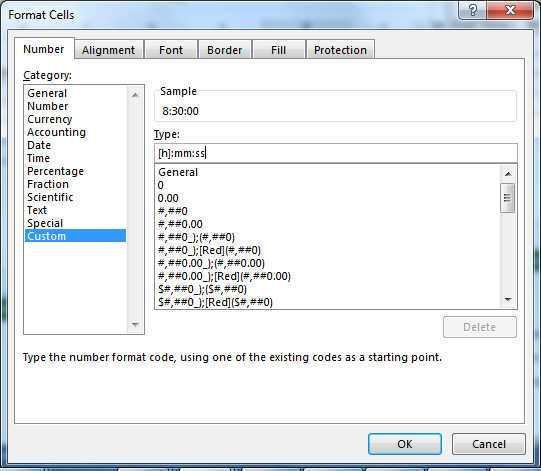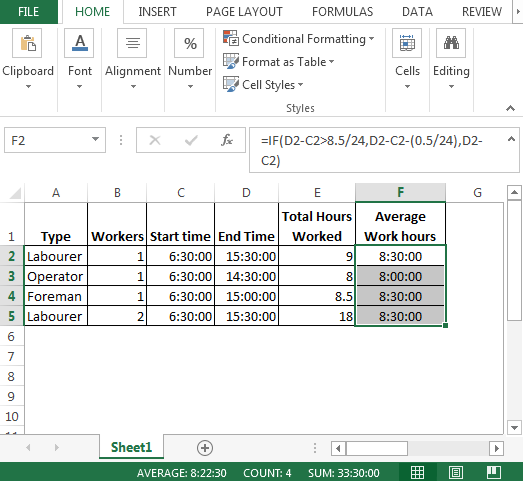# Evaluate Work Hours Excluding Breaks

In case you are evaluating work hours without including break timings then this article is for you. In this article, we will calculate work hours based on their start & end time with a specific condition i.e. if the working hours are less than 8:30 or 8.5 hours then formula should do nothing else formula should deduct 30 minutes.

Question: I need this formula to work over the number of hours worked multiplied by number of workers, and it must be minus 30minutes off if they have worked over 8.5 hours. If they have worked for less than 8.5 hours then result should be produced in form of exact working time of the workers.

Following is the snapshot of data we have:• To calculate “Total Hours Worked”, the formula we are going to use will involve MOD function.
• In cell E2, the formula is
• =(MOD(\$D2-\$C2,1))*24*\$B2• Now, we have calculated “Total Hours Worked”. Let us now see how we can calculate “Average Work Hours”
• In cell F2, the formula is
• =IF(D2-C2>8.5/24,D2-C2-(0.5/24),D2-C2)• Now, we have got the average work hours. Because the format is not what we are looking for so we will do some custom formatting.
• Using CTRL + 1 shortcut key for Format Cells dialog box
• In Number tab itself, click on Custom Category & then select Type
• In Type, enter the hour format as [h]:mm:ss• After converting decimal numbers into proper hourly format, this is how our final output looks.Conclusion: If you have also have prerequisite condition with very few changes then this tutorial will definitely help you & we can evaluate working hours after subtracting break timings.We would love to hear from you, do let us know how we can improve, complement or innovate our work and make it better for you. Write us at info@exceltip.com

## Users are saying about us...

1.useful.

2.